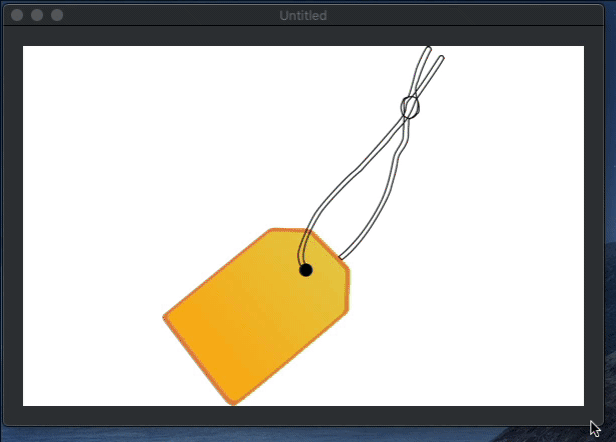Sure you can create a Subclass of any Picture, Canvas or class and write code to center another Picture in its respective Graphics contexts. But taking the class extension approach means that you can reuse the same code for any of these (or any additional class) offering a Graphic context, so you can pass along the Picture you want to center and re-scale (if needed) in its area.

You may already know that in order to create a Method Class Extension, you need to add a new method to a Module. For example, you can create a new Module and name it “Graphic Extensions”.

Then add a new Method to it using the following signature:

• Name: CenterPicture
• Parameters: Extends g As Graphics, Image As Picture
• Scope: GlobalAdd the code that will position and scale the received picture on the Graphic area:

```// Let's check first that the referenced Picture is not nil
If Not (image Is Nil) Then

Var Height, Width As Integer
Var SourceY, SourceX As Integer = 0

//If the Image width and Height just fit inside the Graphic area
//then there is not need to scale it.
If image.Width <= g.Width And image.Height <= g.Height Then

Width = image.Width
Height = image.Height

Else

// Else, let's scale the image when its width is greater
// than its height
If g.Width >= g.Height And image.Width >= image.Height Then

Width = g.Width
Height = (Image.Height * Width) / image.Width

//…recalculating its width if the new height is greater
// than the height of the current Graphics context
If Height > g.Height Then

Height = g.Height
Width = (image.Width*Height)/image.Height

End If

// let's scale the image when its width is less than its height
// but the width of the graphics context is greater than its height
Elseif g.Width >= g.Height And image.Width <= image.Height Then

Height = g.Height
Width = (image.Width * Height) / image.Height

// scale the imagen when the width is greater than its height
// and the width from the Graphics context is less than its height
Elseif g.Width <= g.Height And image.Width >= image.Height Then

Width = g.Width
Height = (image.Height * Width) / image.Width

// lastly, this is the case when both the image and Graphics width
// is less than its respective heights.
Elseif g.Width <= g.Height And image.Width <= image.Height Then

Height = g.Height
Width = (image.Width*Height)/image.Height

// It may be the case that the new calculated width is greater
// than the available width in the Graphics context,
// so we adjust the scale on the max width for the image.
If Width > g.Width Then

Width = g.Width
Height = (image.Height*Width)/image.Width

End If

End If

End If

// Let's calculate the X and Y coordinates in order to
// display the image centered on the Graphics context
sourcex = (g.Width/2) - (Width/2)
sourcey = (g.Height/2) - (Height/2)

// and we draw finally the picture in the Graphic context
// using the new calculated values
g.DrawPicture(image,sourcex,sourcey,Width,Height,0,0,image.Width,Image.Height)

End If```

And that’s all.

From now on, you can call this method from the Paint Event Handler of a Canvas subclass, or the Graphics context of another Picture using:

`g.CenterPicture(mImage) // mImage is a valid instance of a Picture`

Of course, you can go ahead and add other useful method extensions to your own Graphics Extensions module.